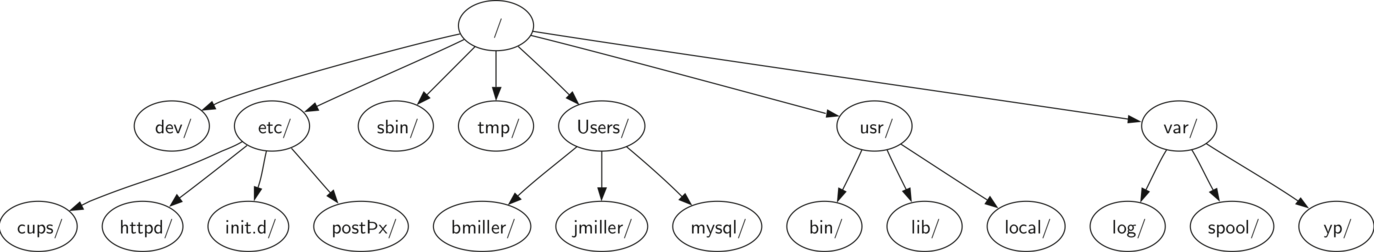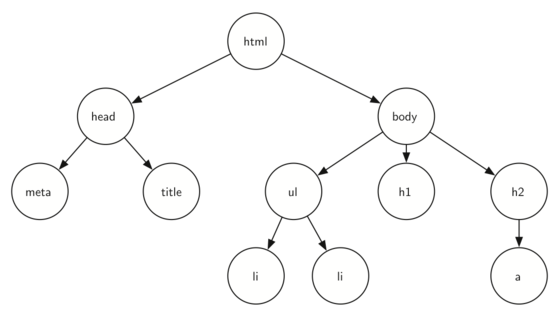# 7.2. Examples of Trees¶

Now that we have studied linear data structures like stacks and queues and have some experience with recursion, we will look at a common data structure called the tree. Trees are used in many areas of computer science, including operating systems, graphics, database systems, and computer networking. Tree data structures have many things in common with their botanical cousins. A tree data structure has a root, branches, and leaves. The difference between a tree in nature and a tree in computer science is that a tree data structure has its root at the top and its leaves on the bottom.

Before we begin our study of tree data structures, let’s look at a few common examples. Our first example of a tree is a classification tree from biology. Figure 1 shows an example of the biological classification of some animals. From this simple example, we can learn about several properties of trees. The first property this example demonstrates is that trees are hierarchical. By hierarchical, we mean that trees are structured in layers with the more general things near the top and the more specific things near the bottom. The top of the hierarchy is the Kingdom, the next layer of the tree (the “children” of the layer above) is the Phylum, then the Class, and so on. However, no matter how deep we go in the classification tree, all the organisms are still animals.Figure 1: Taxonomy of Some Common Animals Shown as a Tree

Notice that you can start at the top of the tree and follow a path made of circles and arrows all the way to the bottom. At each level of the tree we might ask ourselves a question and then follow the path that agrees with our answer. For example we might ask, “Is this animal a Chordate or an Arthropod?” If the answer is “Chordate” then we follow that path and ask, “Is this Chordate a Mammal?” If not, we are stuck (but only in this simplified example). When we are at the Mammal level we ask, “Is this Mammal a Primate or a Carnivore?” We can keep following paths until we get to the very bottom of the tree where we have the common name.

A second property of trees is that all of the children of one node are independent of the children of another node. For example, the Genus Felis has the children Domestica and Leo. The Genus Musca also has a child named Domestica, but it is a different node and is independent of the Domestica child of Felis. This means that we can change the node that is the child of Musca without affecting the child of Felis.

A third property is that each leaf node is unique. We can specify a path from the root of the tree to a leaf that uniquely identifies each species in the animal kingdom; for example, Animalia $$\rightarrow$$ Chordate $$\rightarrow$$ Mammal $$\rightarrow$$ Carnivora $$\rightarrow$$ Felidae $$\rightarrow$$ Felis $$\rightarrow$$ Domestica.

Another example of a tree structure that you probably use every day is a file system. In a file system, directories, or folders, are structured as a tree. Figure 2 illustrates a small part of a Unix file system hierarchy.Figure 2: A Small Part of the Unix File System Hierarchy

The file system tree has much in common with the biological classification tree. You can follow a path from the root to any directory. That path will uniquely identify that subdirectory (and all the files in it). Another important property of trees, derived from their hierarchical nature, is that you can move entire sections of a tree (called a subtree) to a different position in the tree without affecting the lower levels of the hierarchy. For example, we could take the entire subtree staring with /etc/, detach etc/ from the root and reattach it under usr/. This would change the unique pathname to httpd from /etc/httpd to /usr/etc/httpd, but would not affect the contents or any children of the httpd directory.

A final example of a tree is a web page. The following is an example of a simple web page written using HTML. Figure 3 shows the tree that corresponds to each of the HTML tags used to create the page.

<html xmlns="http://www.w3.org/1999/xhtml"
xml:lang="en" lang="en">
<meta http-equiv="Content-Type"
content="text/html; charset=utf-8" />
<title>simple</title>
<body>
<h1>A simple web page</h1>
<ul>
<li>List item one</li>
<li>List item two</li>
</ul>
<h2><a href="http://www.cs.luther.edu">Luther CS </a><h2>
</body>
</html>Figure 3: A Tree Corresponding to the Markup Elements of a Web Page

The HTML source code and the tree accompanying the source illustrate another hierarchy. Notice that each level of the tree corresponds to a level of nesting inside the HTML tags. The first tag in the source is <html> and the last is </html> All the rest of the tags in the page are inside the pair. If you check, you will see that this nesting property is true at all levels of the tree.